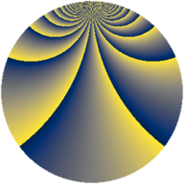# Properties

 Label 507.2.sLevel $507$ Weight $2$ Character orbit 507.s Rep. character $\chi_{507}(5,\cdot)$ Character field $\Q(\zeta_{52})$ Dimension $1392$ Newform subspaces $1$ Sturm bound $121$ Trace bound $0$

# Related objects

## Defining parameters

 Level: $$N$$ $$=$$ $$507 = 3 \cdot 13^{2}$$ Weight: $$k$$ $$=$$ $$2$$ Character orbit: $$[\chi]$$ $$=$$ 507.s (of order $$52$$ and degree $$24$$) Character conductor: $$\operatorname{cond}(\chi)$$ $$=$$ $$507$$ Character field: $$\Q(\zeta_{52})$$ Newform subspaces: $$1$$ Sturm bound: $$121$$ Trace bound: $$0$$

## Dimensions

The following table gives the dimensions of various subspaces of $$M_{2}(507, [\chi])$$.

Total New Old
Modular forms 1488 1488 0
Cusp forms 1392 1392 0
Eisenstein series 96 96 0

## Trace form

 $$1392q - 22q^{3} - 52q^{4} - 22q^{6} - 56q^{7} - 22q^{9} + O(q^{10})$$ $$1392q - 22q^{3} - 52q^{4} - 22q^{6} - 56q^{7} - 22q^{9} - 52q^{10} - 26q^{12} - 44q^{13} - 34q^{15} + 48q^{16} - 34q^{18} - 56q^{19} - 22q^{21} - 96q^{22} - 194q^{24} - 52q^{25} - 34q^{27} - 56q^{28} + 78q^{30} - 32q^{31} - 10q^{33} - 52q^{34} - 26q^{36} - 56q^{37} + 70q^{39} - 36q^{40} - 10q^{42} - 52q^{43} - 140q^{45} - 180q^{46} - 2q^{48} - 52q^{49} - 26q^{51} - 64q^{52} - 22q^{54} + 132q^{55} - 22q^{57} - 60q^{58} - 18q^{60} - 92q^{61} + 108q^{63} - 52q^{64} - 124q^{66} - 240q^{67} - 104q^{69} - 268q^{70} - 2q^{72} - 56q^{73} + 104q^{75} - 48q^{76} - 22q^{78} - 12q^{79} + 2q^{81} + 208q^{82} - 22q^{84} - 52q^{85} - 26q^{87} - 260q^{88} - 26q^{90} - 32q^{91} - 176q^{93} - 240q^{94} + 292q^{96} - 80q^{97} - 58q^{99} + O(q^{100})$$

## Decomposition of $$S_{2}^{\mathrm{new}}(507, [\chi])$$ into newform subspaces

Label Dim. $$A$$ Field CM Traces $q$-expansion
$$a_2$$ $$a_3$$ $$a_5$$ $$a_7$$
507.2.s.a $$1392$$ $$4.048$$ None $$0$$ $$-22$$ $$0$$ $$-56$$